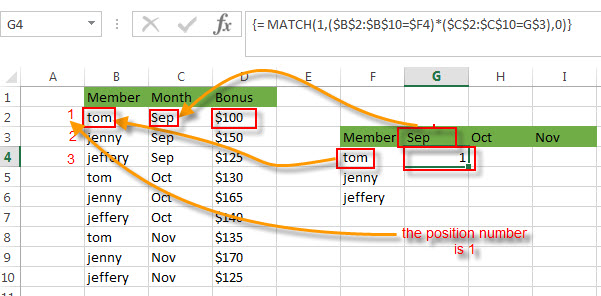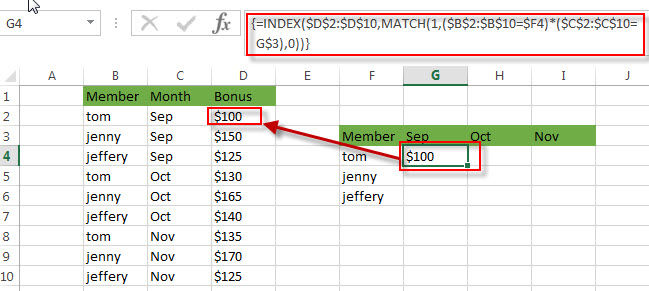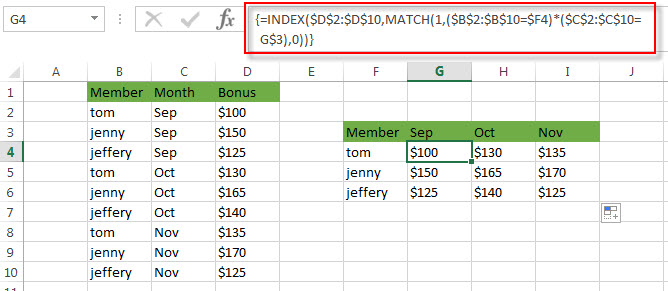# How to Transpose Values Based on the Multiple Lookup Criteria

This post explains that how to transpose values from columns to rows based on the multiple lookup criteria in excel.

In the previous, we talked that how to transpose values from columns to rows using Paste Special Transpose, it’s just rearrange all data in a range and do not apply for any criteria.

## Transpose Values Based on the Multiple Lookup Criteria

If you want to lookup the value with multiple criteria, and then transpose the last results, you can use the INDEX function with the MATCH function to create a new formula.

For example, to transpose the values in both column B and Column C based on the multiple criteria: member’s name is equal to the range B2:B10, and month’s value is equal to the range C2:C10, then extract the bonus value from the range D2:D10. you can use the following array formula:

`=INDEX(\$D\$2:\$D\$10,MATCH(1,(\$B\$2:\$B\$10=\$F4)*(\$C\$2:\$C\$10=G\$3),0))`

Let’s see how the above formula works:

=(\$B\$2:\$B\$10=\$F4)

The above formula will check if each value in the range B2:B10 is equal to the value in Cell F4, if so, return TRUE, otherwise, returns FALSE. So the above formula returns an array result like this:

`{TRUE;FALSE;FALSE;TRUE;FALSE;FALSE;TRUE;FALSE;FALSE}`

=(\$C\$2:\$C\$10=G\$3)

The above formula will check if each month value in the range C2:C10 is equal to the value in Cell G3, if so, return TRUE, otherwise, returns FALSE. So the above formula returns an array result like this:

`{TRUE;TRUE;TRUE;FALSE;FALSE;FALSE;FALSE;FALSE;FALSE}`

=(\$B\$2:\$B\$10=\$F4)*(\$C\$2:\$C\$10=G\$3)

the above formula returns an array result like this:

`{1;0;0;0;0;0;0;0;0}`

= MATCH(1,(\$B\$2:\$B\$10=\$F4)*(\$C\$2:\$C\$10=G\$3),0)

The MATCH function used the above array result(containing one and zero) to find the position of item “1”, it is actually the position of the bonus value that matched the multiple criteria.=INDEX(\$D\$2:\$D\$10,MATCH(1,(\$B\$2:\$B\$10=\$F4)*(\$C\$2:\$C\$10=G\$3),0))

The INDEX function extracts the value based on the position result returned by the above MATCH function. So it returns “\$100”in Cell G4.You can drag the Fill Handler in the Cell G4 to other cells to fill up the entire range G4:I6 as you need.### Related Formulas

• Reverse a List or Range
If you want to reverse a list or range, you can use a combination of the INDEX function, the COUNTA function, the ROW function or ROWS function to create a new formula. you can use the following formula:=INDEX(\$A\$2:\$A\$5,COUNTA(\$A\$2:\$A\$5)-ROWS(\$C\$2:C2)+1)…
• Lookup the Value with Multiple Criteria
If you want to lookup the value with multiple criteria in a range, you can use a combination with the INDEX function and MATCH function to create an array formula…

### Related Functions

• Excel INDEX function
The Excel INDEX function returns a value from a table based on the index (row number and column number)The INDEX function is a build-in function in Microsoft Excel and it is categorized as a Lookup and Reference Function.The syntax of the INDEX function is as below:= INDEX (array, row_num,[column_num])…
• Excel MATCH function
The Excel MATCH function search a value in an array and returns the position of that item.The syntax of the MATCH function is as below:= MATCH  (lookup_value, lookup_array, [match_type])….

Related Posts

Break ties with helper COUNTIF and column

Suppose you got a task to adjust the values that contain the ties; what would be your first attempt to break the ties of the given value? If you are wondering about doing this task manually, let me add that ...

Find the Closest Data to the Data Provided in Excel

In our daily work, we may encounter such an issue that to find the closest value to a certain value. In fact, Excel internal functions can help us solve this problem. In today’s article, we will show you how to ...

Abbreviate Names Or Words in Excel

As an MS Excel user, you might have come across a task where you need to abbreviate different names or words, and there are also possibilities that you might have done this task manually by assuming that there isn't any ...

Convert State Names To Abbreviations

Assume that you got a task to convert the full state's name into the abbreviations in MS Excel, and for doing this task, you might choose to do it manually, which is an acceptable way only if you don't have ...

Filter or Remove Columns

Assume that in MS Excel, you have a table consisting of a few columns consisting of few values, and you want to filter to remove the specified columns from the table. You might take it easy and would prefer to ...

Filter or Extract for Multiple OR Criteria

If you are an valid MS Excel user, you have probably come across a situation where you wanted to filter the data in a separate table with specific criteria. You could do this task manually, which is also acceptable when ...

Filter Multiple Values

In Excel, you can easily filter a table to display only the rows that meet your criteria. This is a quick way to find the information you need without scrolling through all the data. In this post, we'll show you ...

Excel XLOOKUP Function

Excel XLOOKUP Function was added into Excel as a beta feature in August 2019 and is now accessible exclusively in Microsoft 365. (as of July 2021). However, if you fall into this category and often deal with big sets of ...

Extract or Filter Top n values

You might have been through this kind of situation where you need to filter out the top n values from the list having few values, and I am also pretty sure about it that you might have chosen to do ...

Extract Unique Items From A List

This post will guide you how to extract unique itmes from a given list in Microsoft Excel. How to create a newly formula to get unique values from a range cells in Excel. The unique list of items is the ...

Sidebar